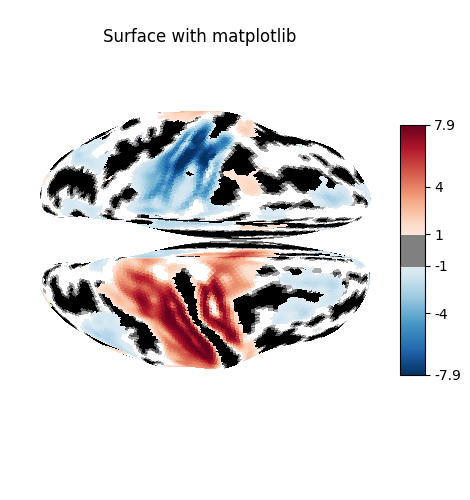# Making a surface plot of a 3D statistical map#

In this example, we will project a 3D statistical map onto a cortical mesh using vol_to_surf, display a surface plot of the projected map using plot_surf_stat_map with different plotting engines, and add contours of regions of interest using plot_surf_contours.

## Get a statistical map#

from nilearn import datasets



## Get a cortical mesh#

fsaverage = datasets.fetch_surf_fsaverage()


Use mesh curvature to display useful anatomical information on inflated meshes

Here, we load the curvature map of the hemisphere under study, and define a surface map whose value for a given vertex is 1 if the curvature is positive, -1 if the curvature is negative.

import numpy as np
from nilearn import surface

curv_right_sign = np.sign(curv_right)


## Sample the 3D data around each node of the mesh#

texture = surface.vol_to_surf(stat_img, fsaverage.pial_right)


## Plot the result#

You can visualize the texture on the surface using the function plot_surf_stat_map which uses matplotlib as the default plotting engine.

from nilearn import plotting

fig = plotting.plot_surf_stat_map(
fsaverage.infl_right, texture, hemi='right',
title='Surface right hemisphere', colorbar=True,
threshold=1., bg_map=curv_right_sign,
)
fig.show()## Interactive plotting with Plotly#

If you have a recent version of Nilearn (>=0.8.2), and if you have plotly installed, you can easily configure plot_surf_stat_map to use plotly instead of matplotlib:

engine = 'plotly'
# If plotly is not installed, use matplotlib
try:
import plotly.graph_objects as go  # noqa: F401
except ImportError:
engine = 'matplotlib'

print(f"Using plotting engine {engine}.")

fig = plotting.plot_surf_stat_map(
fsaverage.infl_right, texture, hemi='right',
title='Surface right hemisphere', colorbar=True,
threshold=1., bg_map=curv_right_sign, bg_on_data=True,
engine=engine  # Specify the plotting engine here
)
fig.show()  # Display the figure as with matplotlib figures

Using plotting engine plotly.
/home/yasmin/nilearn/nilearn/nilearn/plotting/js_plotting_utils.py:81: UserWarning: vmin cannot be chosen when cmap is symmetric
warnings.warn('vmin cannot be chosen when cmap is symmetric')